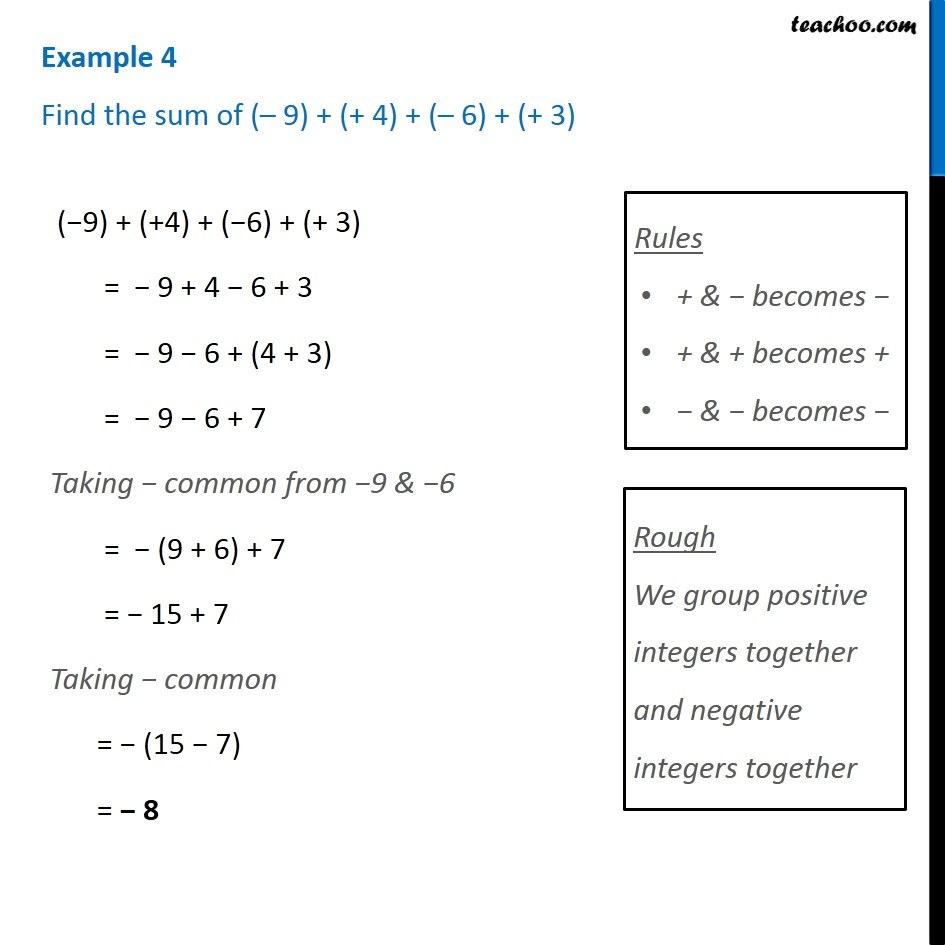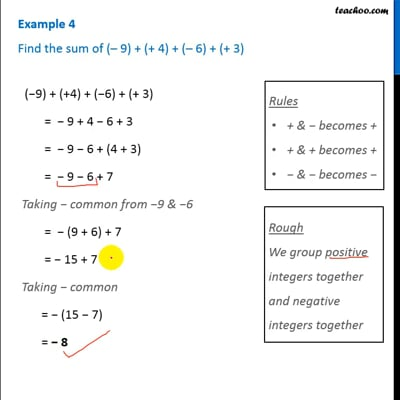Examples

Chapter 6 Class 6 Integers
Serial order wiseThis video is only available for Teachoo black users

Learn in your speed, with individual attention - Teachoo Maths 1-on-1 Class

### Transcript

Example 4 Find the sum of (– 9) + (+ 4) + (– 6) + (+ 3) (−9) + (+4) + (−6) + (+ 3) = − 9 + 4 − 6 + 3 = − 9 − 6 + (4 + 3) = − 9 − 6 + 7 Taking − common from −9 & −6 = − (9 + 6) + 7 = − 15 + 7 Taking − common = − (15 − 7) = − 8 Rules + & − becomes + + & + becomes + − & − becomes − Rough We group positive integers together and negative integers together